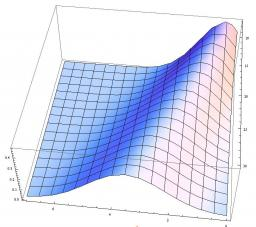# Expression: 4085

Determine the value of x and y in the expression: x! · 10^y = 0.0504.

x =  7
y =  -5

### Step-by-step explanation:Did you find an error or inaccuracy? Feel free to write us. Thank you!

Tips for related online calculators
Do you have a linear equation or system of equations and looking for its solution? Or do you have a quadratic equation?